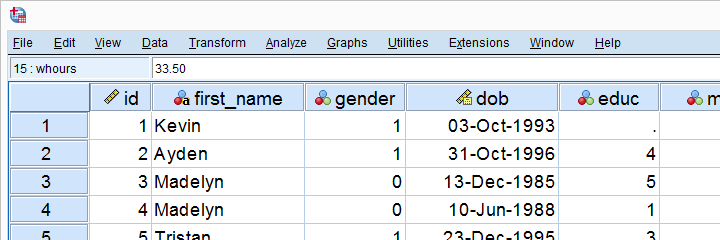SPSS TUTORIALS BASICS ANOVA REGRESSION FACTOR CORRELATION

# SPSS Beginners Tutorials## Introduction to SPSS

### SPSS – What Is It?

IBM SPSS Statistics (or “SPSS” for short) is super easy software for editing and analyzing data.

This tutorial presents a quick overview of what SPSS looks like and how it basically works.

### SPSS Data Editor Window

SPSS’ main window is the data editor. It shows our data so we can visually inspect it.

This tutorial explains how the data editor works: we'll walk you through its main parts and point out some handy tips & tricks.

### SPSS Syntax Introduction

SPSS syntax is computer code used by SPSS for analyzing data, editing data, running statistical tests and more.

Using SPSS syntax is super easy and saves tons of time and effort. This tutorial quickly gets you started!

### SPSS Output – Basics, Tips & Tricks

SPSS’ output window shows the tables, charts and statistical tests you run while analyzing your data.

This tutorial walks you through some basics such as exporting tables and charts to WORD or Excel. We'll also point out some important tricks such as batch editing and styling tables and charts.

## Normality Tests in SPSS

### SPSS Shapiro-Wilk Test – Quick Tutorial with Example

The Shapiro-Wilk test examines if a variable is normally distributed in a population. This assumption is required by some statistical tests such as t-tests and ANOVA.

The SW-test is an alternative for the Kolmogorov-Smirnov test. This tutorial shows how to run and interpret it in SPSS.

### SPSS Kolmogorov-Smirnov Test for Normality

The Kolmogorov-Smirnov test examines if a variable is normally distributed in some population.

This “normality assumption” is required for t-tests, ANOVA and many other tests. This tutorial shows how to run and interpret a Kolmogorov-Smirnov test in SPSS with some simple examples.

## SPSS - Popular Tutorials

### SPSS Missing Values Tutorial

In SPSS, missing values refer to

• system missing values: values that are absent from the data;
• user missing values: values that are present in the data but must be excluded from analyses.

We'll quickly walk you through both types. We'll also show how to detect, set and deal with missing values in SPSS.

### SPSS Factor Analysis – Beginners Tutorial

Factor analysis examines which variables in your data measure which underlying factors.

This tutorial illustrates the ideas behind factor analysis with a simple step-by-step example in SPSS.

### How to Run Levene’s Test in SPSS?

Levene’s test examines if 2+ populations have equal variances on some variable.

This condition -known as the homogeneity of variance assumption- is required by t-tests and ANOVA.

So how to run and interpret this test in SPSS? This simple tutorial quickly walks you through.

### SPSS IF – A Quick Tutorial

In SPSS, IF computes a new or existing variable but for a selection of cases only.

For example: IF(GENDER = 0) SCORE = MEAN(Q1 TO Q5). computes “score” as the mean over variables Q1 to Q5 but only for cases whose gender is 0 (female).

## Must-Know Statistics

### Effect Size – A Quick Guide

Effect size is an interpretable number that quantifies the difference between data and some hypothesis.

Effect size measures are useful for comparing effects across and within studies. This tutorial helps you to choose, obtain and interpret an effect size for each major statistical procedure.

### Cohen’s D – Effect Size for T-Test

Cohen’s D is the effect size measure of choice for t-tests.

This simple tutorial quickly walks you through

• rules of thumb for small, medium and large effects;
• formulas for computing Cohen’s D and;
• software options for obtaining it.

### Percentiles – Quick Introduction & Examples

The nth percentile is the value that separates the lowest n% from all other values.

Example: the 10th percentile for body weight is 60 kilos. This means that 10% of all people weigh less than 60 kilos and 90% of people weigh more.

Simple tutorial with examples in Excel & SPSS and (interpolation) formulas.

### What Does “Statistical Significance” Mean?

Statistical significance is roughly the probability of finding your data under some null hypothesis.

If this probability (or “p”) is low -usually p < 0.05- then your data contradict your null hypothesis. In this case, you conclude that the hypothesis is not true.

# Tell us what you think!

*Required field. Your comment will show up after approval from a moderator.

# THIS TUTORIAL HAS 115 COMMENTS:

•### By Rita on April 15th, 2023

You saved my life!! THANK YOU!!!

•### By Ekram Ayman on June 7th, 2023

thanks

•### By ajuna brian on June 19th, 2023

so good

•### By Laila on August 10th, 2023

excellent SPSS course

•### By Serious Black on September 7th, 2023

that is a good software for data analysis for survey but it is not easy to understand and use